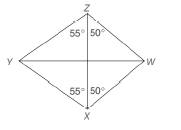Chapter 4.2, Problem 8E### Elementary Geometry for College St...

6th Edition
Daniel C. Alexander + 1 other
ISBN: 9781285195698

#### Solutions

Chapter
Section### Elementary Geometry for College St...

6th Edition
Daniel C. Alexander + 1 other
ISBN: 9781285195698
Textbook Problem
1 views

# In quadrilateral W X Y Z , the measures of selected angles are shown.a) What type of quadrilateral is W X Y Z ?b) Which diagonal of the quadrilateral has the greater length?To determine

The type of the quadrilateral WXYZ.

Explanation

Given,

From the above given figure

We have

mYZX=mYZX=55°,

mWZX=mWXZ=50°.

Thus,

mYZW=mYZX+mWZX

=55°+50°

To determine

b)

The diagonal of the quadrilateral that has greater length.

### Still sussing out bartleby?

Check out a sample textbook solution.

See a sample solution

#### The Solution to Your Study Problems

Bartleby provides explanations to thousands of textbook problems written by our experts, many with advanced degrees!

Get Started

#### (2, 5]

Applied Calculus for the Managerial, Life, and Social Sciences: A Brief Approach

#### Evaluate the expression sin Exercises 116. (2)3

Finite Mathematics and Applied Calculus (MindTap Course List)

#### An integral for the solid obtained by rotating the region at the right about the y-axis is:

Study Guide for Stewart's Single Variable Calculus: Early Transcendentals, 8th